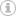# How to add days, months, and years to a date in JavaScriptSeveral years ago I wrote a post about how to add days, months, and years in JavaScript, and it was a quick-and-dirty workaround for a project and I didn’t pay much attention to value checking when writing the JavaScript function. For example, if the old date is 07/19/2017 and you need to know what date it is after adding 25 days, the JavaScript function will throw an error because 07/44/2017 is not a valid date string. Similarly, if you add 6 months on the old date (07/19/2017) you will get an error too, instead of getting the correct date 01/19/2018. I knew this issue but never had time to address it. After being asked by a reader of the old post, I decided to re-write the JavaScript function to better handle situations like aforementioned.

Before I paste the JavaScript function, let me explain the problem and highlight some important aspects of the problem.

The function is called AddDate(oldDate, offset, offsetType), the parameter “oldDate” is the starting date, the “offset” is the number of days, months, or years that being added to the “oldDate”, and the “offsetType” tells the function if the unit of the “offset” is day, month, year, or even hour. Let’s go over each type of the offset and explain how to calculate the new date.

1. offsetType = Year

It is the easiest scenario for calculating a new date. First, we will strip off the year portion from the old date by using Date.getFullYear() function, then we will add the offset to the year to make a new date string which will be used to create the new date.``` var year = parseInt(oldDate.getFullYear()); newDate = new Date(year + offset, month, date, hour); ```

2. offsetType = Month

This scenario is a little tricky because if the month portion of the new date (after adding the offset) is more than 12, then we need to make sure to calculate the correct year and month. For instance, if the month portion of the old date is 05 and the offset is 9, then we need to add one year to the new date and the new month portion will be 02, instead of 14. Here is the algorithm for handling this scenario:

a) if the offset is less than 12, then the integer portion of (month + offset)/12 will be the offset for the new year portion, and (month + offset)%12 will be the offset of the new month portion

b) if the offset is greater than or equal to 12, then the integer portion of offset/12 will be the offset for the new year portion, and (month%12 + offset%12) will be the offset of the new month portion

Here is the code snippet:``` var year = parseInt(oldDate.getFullYear()); var month = parseInt(oldDate.getMonth()); var yearOffset = 0; var monthOffset = 0; if (offset < 12) { yearOffset = Math.floor((month + offset)/12); monthOffset = (month + offset)%12; } else { yearOffset = Math.floor(offset/12); monthOffset = month%12 + offset%12; } newDate = new Date(year + yearOffset, month + monthOffset, date, hour); ```

3. offsetType = Day

This scenario is even trickier because the offset may affect the month portion and the year portion of the date after adding the offset to the day portion. For example, if the current date is 07/20/2017 and the offset is 65, then it will affect the month portion of the new date. But if the offset is 165, then it will affect both the month portion and the year portion of the new date. Luckily, the JavaScript Date object has a method called getTime() which returns the number of milliseconds since 01/01/1970, so we can first calculate the number of milliseconds of the old date, then add the milliseconds of the offset to create a new date. Here is the code snippet:``` var o = oldDate.getTime(); var n = o + offset * 24 * 3600 * 1000; newDate = new Date(n); ```
4. offsetType = Hour

We can use the same way as above to calculate the new date and the only difference will be the number of milliseconds of the offset:``` var o = oldDate.getTi(); var n = o + offset * 3600 * 1000; newDate = new Date(n); ```

5. Put Everything Together

After putting all code snippets, here is the final version of the JavaScript function:``` function AddDate(oldDate, offset, offsetType) { var year = parseInt(oldDate.getFullYear()); var month = parseInt(oldDate.getMonth()); var date = parseInt(oldDate.getDate()); var hour = parseInt(oldDate.getHours()); var newDate; switch (offsetType) { case "Y": case "y": newDate = new Date(year + offset, month, date, hour); break;```
``` case "M": case "m": var yearOffset = 0; var monthOffset = 0; if (offset <12) { yearOffset = Math.floor((month + offset)/12); monthOffset = (month + offset)%12; } else { yearOffset = Math.floor(offset/12); monthOffset = month%12 + offset%12; } newDate = new Date(year + yearOffset, month + monthOffset, date, hour); break; ```
``` case "D": case "d": var o = oldDate.getTime(); var n = o + offset * 24 * 3600 * 1000; newDate = new Date(n); break;```
``` case "H": case "h": var o = oldDate.getTi(); var n = o + offset * 3600 * 1000; newDate = new Date(n); break;`````` default: newDate = new Date(year + offset, month, date, hour); } return newDate; } ```
Please let me know if this function helped you or if you have questions.0 Followers

Most reacted comment
4 Comment authorsRecent comment authors
Subscribe
Notify ofGuest
JayGuest
Ewgenij Sokolovski

You can just usewpDiscuz